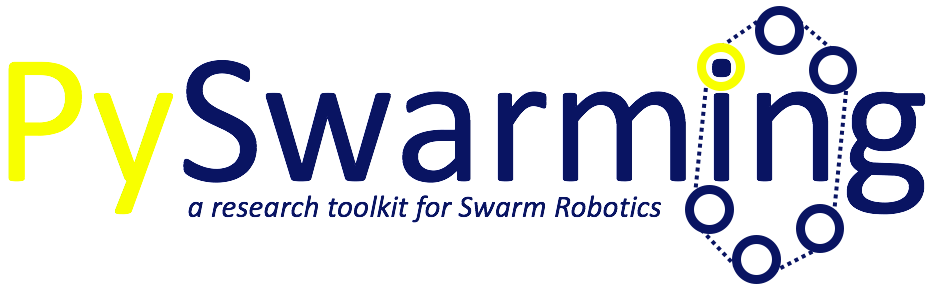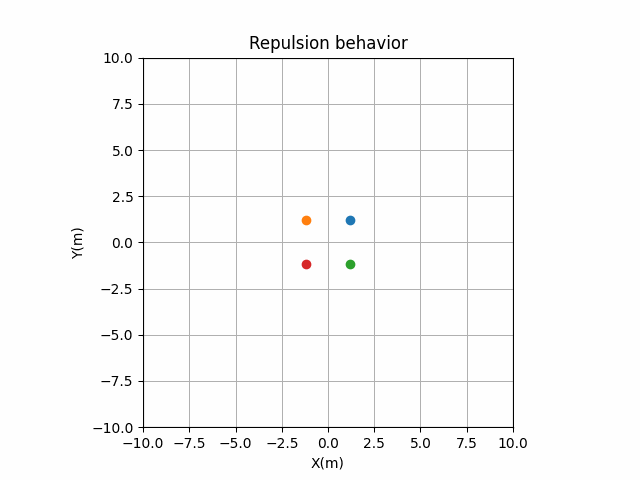# Welcome to pyswarming’s documentation!## Introduction

`pyswarming` is a research toolkit for Swarm Robotics.

The package is currently maintained by @mrsonandrade.

## Install

You can install `pyswarming` from PyPI using pip (Recommended):

```pip install pyswarming
```

## Dependencies

`pyswarming`’s dependencies are: `numpy`.

## Algorithms covered

This library includes the following algorithms to be used in swarm robotics:

• Collision Avoidance: the robot stay away from neighbors in the vicinity 3;

• Attraction and Alignment: the robot become attracted and aligned 3;

• Preferred Direction: the robot has preference to move toward a preset direction 3;

• Modified Attraction and Alignment: the robot become attracted and aligned by considering a “social importance” factor 4;

• Perimeter Defense: the robots maximize the perimeter covered in a an unknown environment 5;

• Environment Exploration: the robots provide a spatial coverage 5;

• Aggregation: makes all the individuals aggregate collectively 6;

• Alignment: the collective performs heading consensus 6;

• Geofencing: attract the robots towards the area A 6;

• Repulsion: makes all the individuals repulse collectively 6;

• Target: the robot goes to an specific target location 6;

References

1

Vicsek T, Czirók A, Ben-Jacob E, Cohen I, Shochet O. Novel Type of Phase Transition in a System of Self-Driven Particles. Phys Rev Lett 1995;75:1226–9. https://doi.org/10.1103/PhysRevLett.75.1226.

2

Jadbabaie A, Jie Lin, Morse AS. Coordination of groups of mobile autonomous agents using nearest neighbor rules. IEEE Trans Automat Contr 2003;48:988–1001. https://doi.org/10.1109/TAC.2003.812781.

3(1,2,3)

Couzin ID, Krause J, Franks NR, Levin SA. Effective leadership and decision-making in animal groups on the move. Nature 2005;433:513–6. https://doi.org/10.1038/nature03236.

4

Freeman R, Biro D. Modelling Group Navigation: Dominance and Democracy in Homing Pigeons. J Navigation 2009;62:33–40. https://doi.org/10.1017/S0373463308005080.

5(1,2,3)

Chamanbaz M, Mateo D, Zoss BM, Tokić G, Wilhelm E, Bouffanais R, et al. Swarm-Enabling Technology for Multi-Robot Systems. Front Robot AI 2017;4. https://doi.org/10.3389/frobt.2017.00012.

6(1,2,3,4,5)

Zoss BM, Mateo D, Kuan YK, Tokić G, Chamanbaz M, Goh L, et al. Distributed system of autonomous buoys for scalable deployment and monitoring of large waterbodies. Auton Robot 2018;42:1669–89. https://doi.org/10.1007/s10514-018-9702-0.

## Examples

Considering a swarm of robots, they can show different behaviors by using `pyswarming`. The following codes are simplified implementations, for detailed ones, see the Examples folder.:

```import pyswarming.behaviors as ps
import numpy as np
```

### Target

```# define the robot (x, y, z) position
r_i = np.asarray([0., 0., 0.])

# set the robot linear velocity
s_i = 1.0

# define a target (x, y, z) position
T = np.asarray([8., 8., 0.])

for t in range(15):

# print the robot (x, y, z) position
print(r_i)

# update the robot (x, y, z) position
r_i += s_i*ps.target(r_i, T)
```### Aggregation

```# define each robot (x, y, z) position
r = np.asarray([[8., 8., 0.],
[-8., 8., 0.],
[8., -8., 0.],
[-8., -8., 0.]])

# set the robot linear velocity
s_i = 1.0

for t in range(15):

# print the robot (x, y, z) positions
print(r)

# update the robot (x, y, z) positions
for r_ind in range(len(r)):
r_i = r[r_ind]
r_j = np.delete(r, np.array([r_ind]), axis=0)
r[r_ind] += s_i*ps.aggregation(r_i, r_j)
```### Repulsion

```# define each robot (x, y, z) position
r = np.asarray([[1., 1., 0.],
[-1., 1., 0.],
[1., -1., 0.],
[-1., -1., 0.]])

# set the robot linear velocity
s_i = 1.0

for t in range(15):

# print the robot (x, y, z) positions
print(r)

# update the robot (x, y, z) positions
for r_ind in range(len(r)):
r_i = r[r_ind]
r_j = np.delete(r, np.array([r_ind]), axis=0)
r[r_ind] += s_i*ps.repulsion(r_i, r_j, 3.0)
```### Aggregation + Repulsion

```# define each robot (x, y, z) position
r = np.asarray([[8., 8., 0.],
[-8., 8., 0.],
[8., -8., 0.],
[-8., -8., 0.]])

# set the robot linear velocity
s_i = 1.0

for t in range(15):

# print the robot (x, y, z) positions
print(r)

# update the robot (x, y, z) positions
for r_ind in range(len(r)):
r_i = r[r_ind]
r_j = np.delete(r, np.array([r_ind]), axis=0)
r[r_ind] += s_i*(ps.aggregation(r_i, r_j) + ps.repulsion(r_i, r_j, 5.0))
```